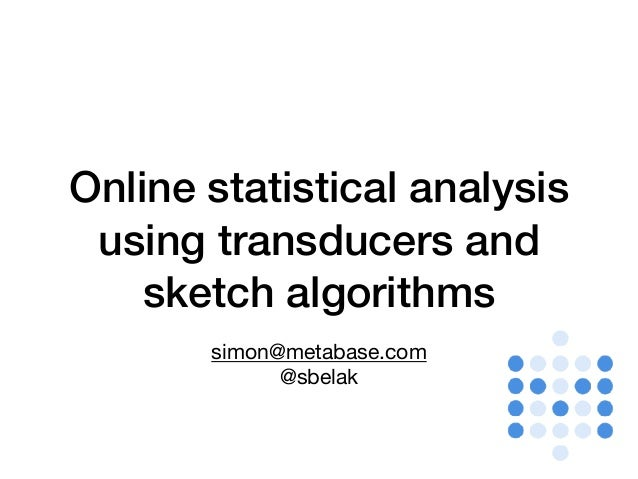Successfully reported this slideshow.Upcoming SlideShare
×

# Online statistical analysis using transducers and sketch algorithms

284 views

Published on

Online statistical analysis using transducers and sketch algorithms. Don’t know what either is? You are going to learn something very cool (and perspective-changing) then. Know them, but want an experience report? Got you covered, fam.

Published in: Data & Analytics
• Full Name
Comment goes here.

Are you sure you want to Yes No• Be the first to comment

• Be the first to like this

### Online statistical analysis using transducers and sketch algorithms

1. 1. Online statistical analysis using transducers and sketch algorithms simon@metabase.com @sbelak
2. 2. Metabase ❤  github.com/metabase/metabase • Open source analytics tool • Building a “data scientist in a box” • Hundreds to billions of rows • Some DBs optimised for analytics, some not
3. 3. Transducers at a glance • Transducers decomplect recursion mechanism, transformation, building the output, and access mechanism            • 3 user-facing “protocols”: xf, transdcucer, and CollReduce
4. 4. xf and transducer
5. 5. Composing transducers 1. comp xfs    2. xf and transducer 3. github.com/henrygarner/redux  post-complete fuse
6. 6. On-line/streaming analysis
7. 7. Many batch algorithms can be turned into online ones Parallelize independent computations Find a recursive relation
8. 8. github.com/MastodonC/kixi.stats • Count • (Arithmetic) mean • Geometric mean • Harmonic mean • Median • Variance • Interquartile range • Standard deviation • Standard error • Skewness • Kurtosis • Covariance • Covariance matrix • Correlation • Correlation matrix • Simple linear regression • Standard error of the mean • Standard error of the estimate • Standard error of the prediction • …
9. 9. Single-pass analysis
10. 10. Using transducers is worth it for the composition alone
11. 11. Annoyances • Can only transduce one coll at a time • Always have to pass in an xf • Having functions that return a transducer or not is error prone
12. 12. Sketch algorithms
13. 13. Idea: summarise your data with some data structure and query that
14. 14. Histograms
15. 15. Histogram construction 1. Pick a number of buckets K 2. For each incoming value: 1. If a bucket for it exists, increment it 2. else, add a new bucket with count = 1 3. If there are > K buckets, ﬁnd the two most adjacent buckets and merge them
16. 16. Nice property: merge
17. 17. Estimating values • Assume the bin mean in also its median • Do weighted interpolations • Often we can be precise up to the two bounding buckets
18. 18. Nice property II:   decouples data collection from computation
19. 19. github.com/bigmlcom/histogram
20. 20. Aside: transducers are a good way to wrap Java/ imperative construction
21. 21. Having distributions readily available is great
22. 22. Sampling trick
23. 23. Time slices
24. 24. What about categorical data?
25. 25. Count–min sketch
26. 26. Often approximations are good enough